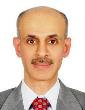#### Hesham K. Alfares

King Fahd University of Petroleum & Minerals, Saudi Arabia

### Biography

Hesham Alfares is professor in the Systems Engineering Department at King Fahd University of Petroleum & Minerals, in Dhahran, Saudi Arabia. He obtained a BS in electrical & Computer Engineering from the University of California, Santa Barbara, an MS in Industrial Engineering from the University of Pittsburgh, and a PhD in Industrial Engineering from Arizona State University.

### Abstract

This paper presents two types of production-inventory systems with stock-level-dependent demand, time-dependent holding cost, and order size-dependent purchase cost. By incorporating these and other unique and realistic considerations, the two systems eliminate several shortcomings of traditional production-inventory systems such as the economic production quantity (EPQ) model. In general, traditional models assume a constant demand rate, a constant unit holding cost, and a constant unit purchase cost. Moreover, traditional models usually aim to minimize the total cost, and they assume the starting and ending inventory levels to be zero. In the systems proposed in this paper, the simplifications and limitations of traditional models are replaced by applicable and realistic assumptions. First, the demand rate is assumed to be an increasing power function of the instantaneous inventory level. Second, the unit holding cost is assumed to be an increasing step function of the storage duration. Third, the unit purchase cost is assumed to be a decreasing step function of the production lot size. An all-units quantity discount scheme is assumed, in which the discounted price applies to all the units in the production lot. Fourth, the objective of the system is to increase the overall profit. Since the demand is not constant, the revenue is not constant, and therefore minimizing the cost is not equivalent to maximizing the profit. Finally, the starting/ending inventory level is assumed to be a decision variable whose value is optimally determined by the model. Clearly, a higher starting/ending inventory level increases the holding cost, but leads to higher demand and greater revenue. The model determines the optimum value of the starting/ending inventory level that provides the best cost-benefit balance and leads to the maximum profit. Two types of holding cost increase with longer storage durations are considered: retroactive and incremental. Retroactive increase means the (highest) holding cost of the latest storage time interval applies to all storage periods. Incremental increase means that a different holding cost is applied to each storage time interval. For each of the two types, a mathematical optimization model is formulated, and an efficient, nonlinear programming-based, technique is developed to find the optimum solution.